## 3.4 Orthogonal basis expansions

### 3.4.1 Polynomial expansions

Degree 19 polynomial fitted to the temperature data.We can extract the model matrix from the lm-object.

intercept <- rep(1/sqrt(n), n)  # To make intercept column have norm one
polylm <- lm(Temperature ~ intercept + poly(Year, 19) - 1, data = Nuuk_year)
Phi <- model.matrix(polylm)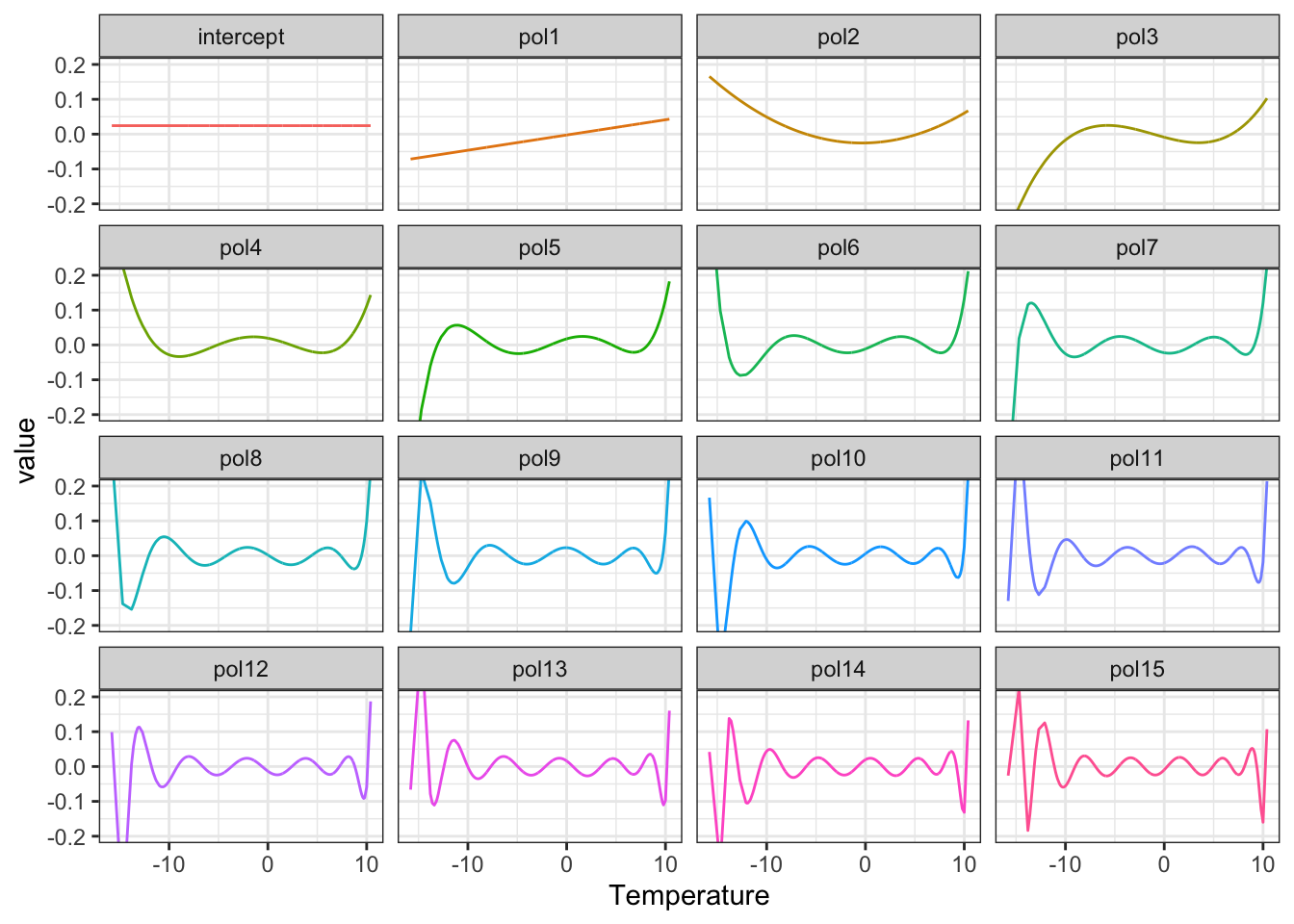Figure 3.10: The model matrix columns as functions

The model matrix is (almost) orthogonal, and estimation becomes quite simple. With an orthogonal model matrix the normal equation reduces to the estimate $\hat{\beta} = \Phi^T Y$ since $$\Phi^T \Phi = I$$. The predicted (or fitted) values are $$\Phi \Phi^T Y$$ with smoother matrix $$\mathbf{S} = \Phi \Phi^T$$ being a projection.

(t(Phi) %*% Nuuk_year$Temperature)[1:10, 1] ## intercept poly(Year, 19)1 poly(Year, 19)2 poly(Year, 19)3 poly(Year, 19)4 poly(Year, 19)5 ## -17.2469646 4.9002430 -1.7968913 0.8175400 5.9668689 1.4265091 ## poly(Year, 19)6 poly(Year, 19)7 poly(Year, 19)8 poly(Year, 19)9 ## -1.9258864 -0.2523581 -2.1355117 -0.8046267 coef(polylm)[1:10] ## intercept poly(Year, 19)1 poly(Year, 19)2 poly(Year, 19)3 poly(Year, 19)4 poly(Year, 19)5 ## -17.2469646 4.9002430 -1.7968913 0.8175400 5.9668689 1.4265091 ## poly(Year, 19)6 poly(Year, 19)7 poly(Year, 19)8 poly(Year, 19)9 ## -1.9258864 -0.2523581 -2.1355117 -0.8046267 With homogeneous variance $\hat{\beta}_i \overset{\text{approx}}{\sim} \mathcal{N}(\beta_i, \sigma^2),$ and for $$\beta_i = 0$$ we have $$P(|\hat{\beta}_i| \geq 1.96\sigma) \simeq 0.05.$$ Thresholding: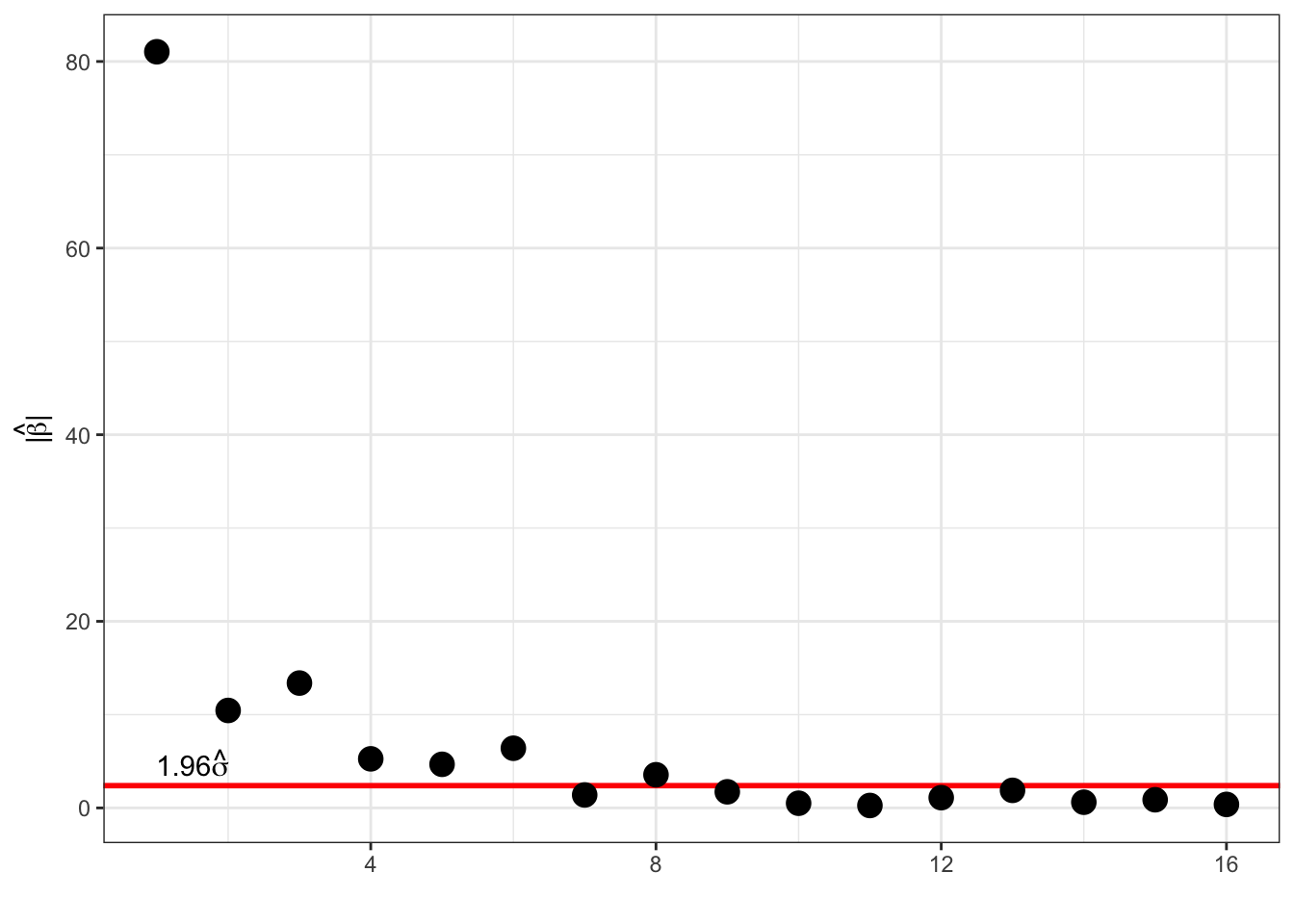Figure 3.11: Polynomial fit using all 19 basis functions (blue) and using a degree 5 polynomial (red). ### 3.4.2 Fourier expansions Introducing $x_{k,m} = \frac{1}{\sqrt{n}} e^{2 \pi i k m / n},$ then $\sum_{k=0}^{n-1} |x_{k,m}|^2 = 1$ and for $$m_1 \neq m_2$$ $\sum_{k=0}^{n-1} x_{k,m_1}\overline{x_{k,m_2}} = 0$ Thus $$\Phi = (x_{k,m})_{k,m}$$ is an $$n \times n$$ unitary matrix; $\Phi^*\Phi = I$ where $$\Phi^*$$ is the conjugate transposed of $$\Phi$$. $$\hat{\beta} = \Phi^* y$$ is the discrete Fourier transform of $$y$$. It is the basis coefficients in the orthonormal basis given by $$\Phi$$; $y_k = \frac{1}{\sqrt{n}} \sum_{m=0}^{n-1} \hat{\beta}_m e^{2 \pi i k m / n}$ or $$y = \Phi \hat{\beta}.$$ Phi <- outer( 0:(n - 1), 0:(n - 1), function(k, m) exp(2 * pi * 1i * (k * m) / n) / sqrt(n) ) The matrix $$\Phi$$ generates an interesting pattern.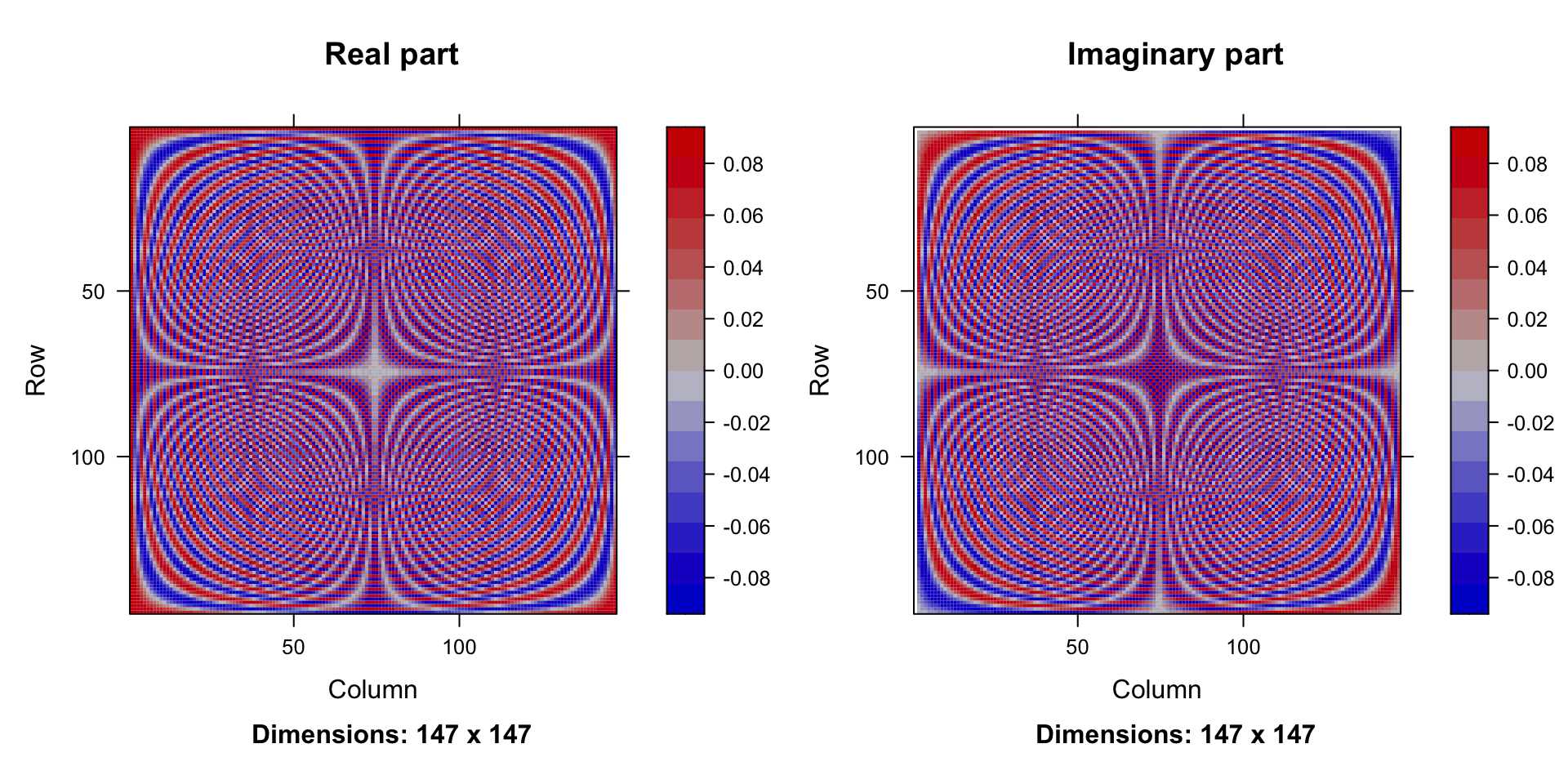Columns in the matrix $$\Phi$$: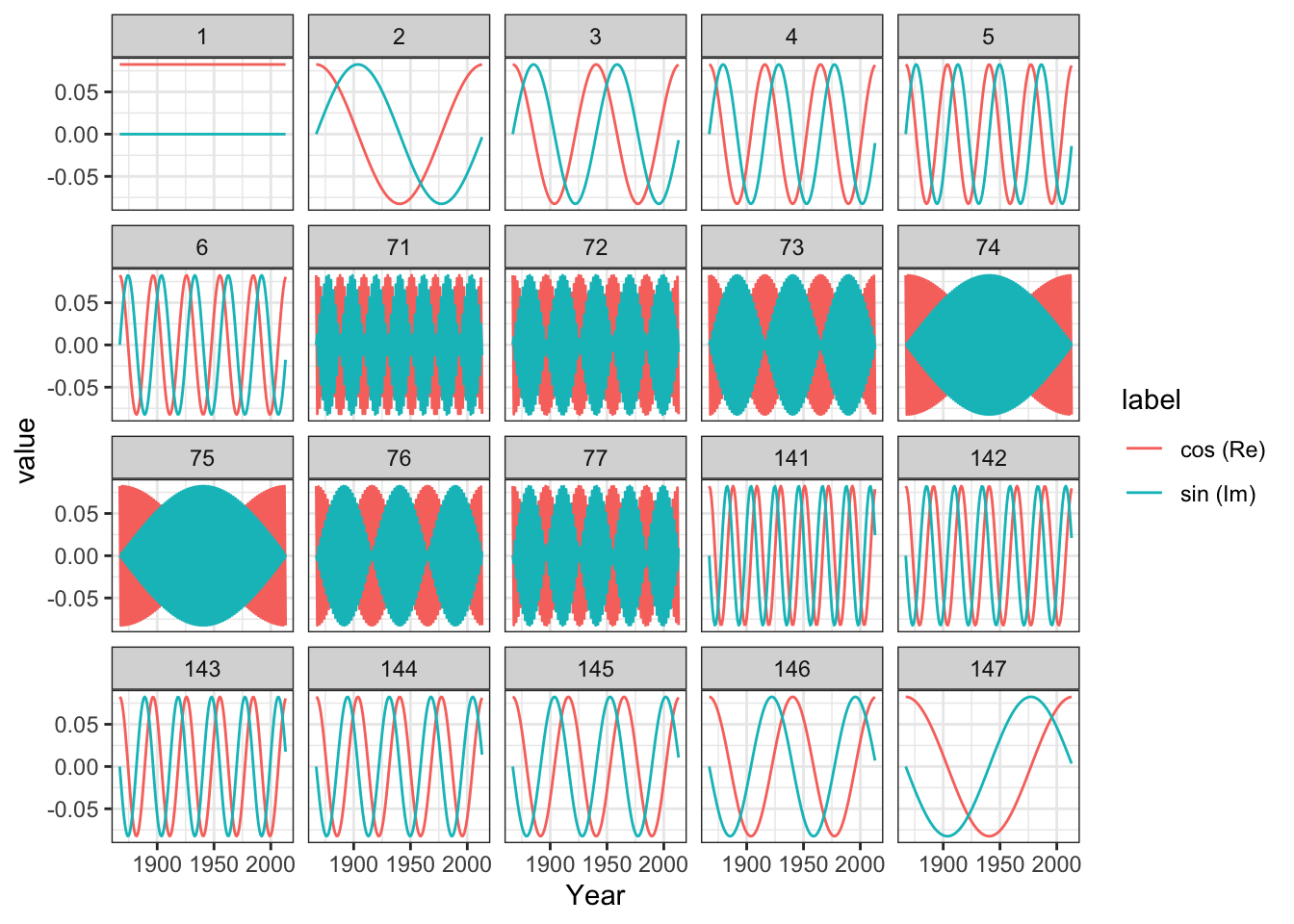We can estimate by matrix multiplication betahat <- Conj(t(Phi)) %*% Nuuk_year$Temperature # t(Phi) = Phi for Fourier bases
betahat[c(1, 2:4, 73, n:(n - 2))]
##  -17.2469646+0.0000000i  -2.4642887+2.3871189i   3.5481329+0.9099226i   1.6721444+0.7413580i
##    0.0321232+0.7089991i  -2.4642887-2.3871189i   3.5481329-0.9099226i   1.6721444-0.7413580i

For real $$y$$ it holds that $$\hat{\beta}_0$$ is real, and the symmetry $\hat{\beta}_{n-m} = \hat{\beta}_m^*$ holds for $$m = 1, \ldots, n - 1$$. (For $$n$$ even, $$\hat{\beta}_{n/2}$$ is real too).

Modulus distribution:

Note that for $$m \neq 0, n/2$$, $$\beta_m = 0$$ and $$y \sim \mathcal{N}(\Phi\beta, \sigma^2 I_n)$$ then
$(\mathrm{Re}(\hat{\beta}_m), \mathrm{Im}(\hat{\beta}_m))^T \sim \mathcal{N}\left(0, \frac{\sigma^2}{2} I_2\right),$

hence $|\hat{\beta}_m|^2 = \mathrm{Re}(\hat{\beta}_m)^2 + \mathrm{Im}(\hat{\beta}_m)^2 \sim \frac{\sigma^2}{2} \chi^2_2,$ that is, $$P(|\hat{\beta}_m| \geq 1.73 \sigma) = 0.05.$$ There is a clear case of multiple testing if we use this threshold at face value, and we would expect around $$0.05 \times n/2$$ false positive if there is no signal at all. Lowering the probability using the Bonferroni correction yields a threshold of around $$2.7 \sigma$$ instead.

Thresholding Fourier: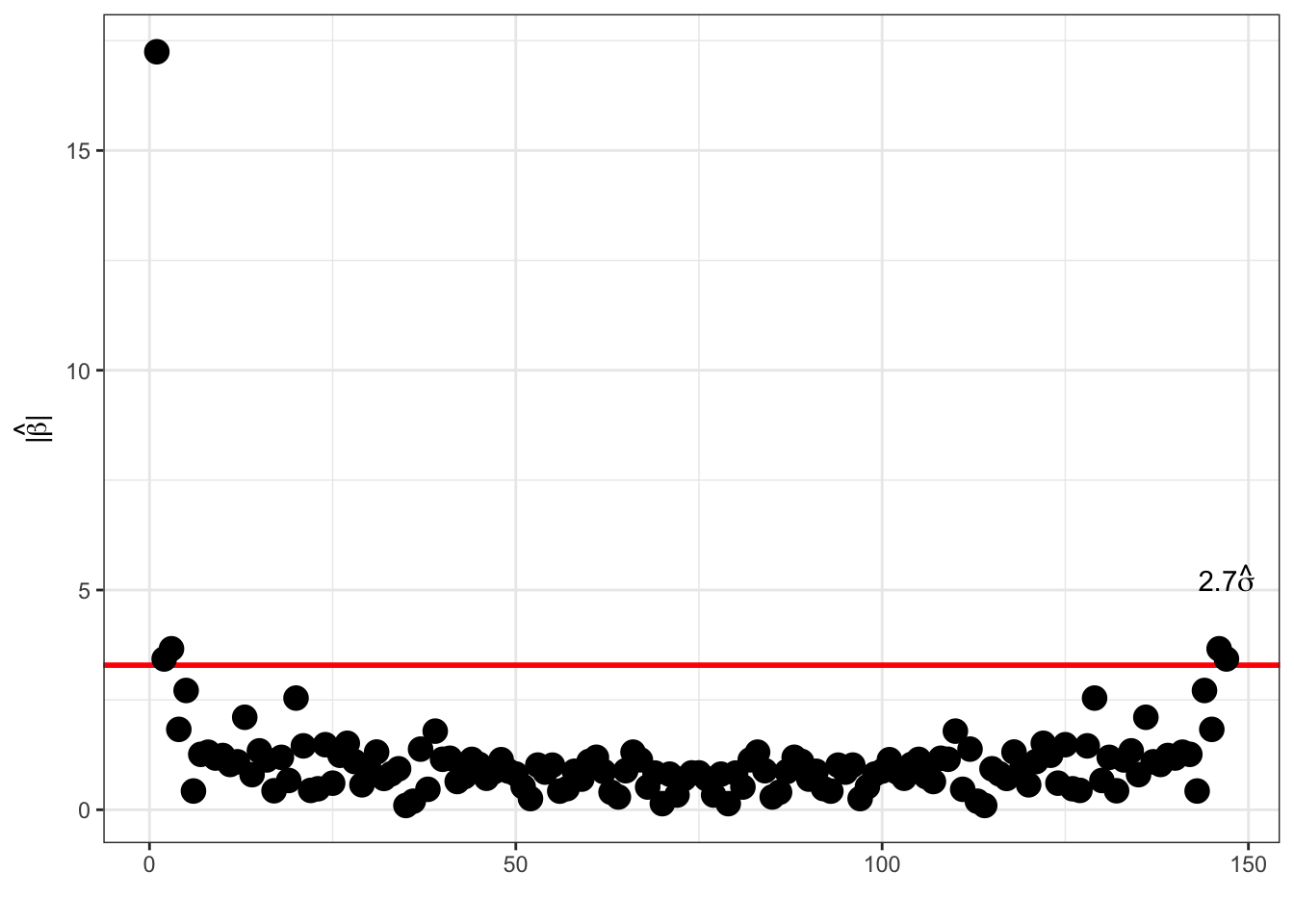The coefficients are not independent (remember the symmetry), and one can alternatively consider $\hat{\gamma}_m = \sqrt{2} \mathrm{Re}(\hat{\beta}_m) \quad \text{and} \quad \hat{\gamma}_{n' + m} = - \sqrt{2} \mathrm{Im}(\hat{\beta}_m)$ for $$1 \leq m < n / 2$$. Here $$n' = \lfloor n / 2 \rfloor$$. Here, $$\hat{\gamma}_0 = \hat{\beta}_0$$, and $$\hat{\gamma}_{n/2} = \hat{\beta}_{n/2}$$ for $$n$$ even.

These coefficients are the coefficients in a real cosine, $$\sqrt{2} \cos(2\pi k m / n)$$, and sine, $$\sqrt{2} \sin(2\pi k m / n)$$, basis expansion, and they are i.i.d. $$\mathcal{N}(0, \sigma^2)$$ distributed.

Thresholding Fourier: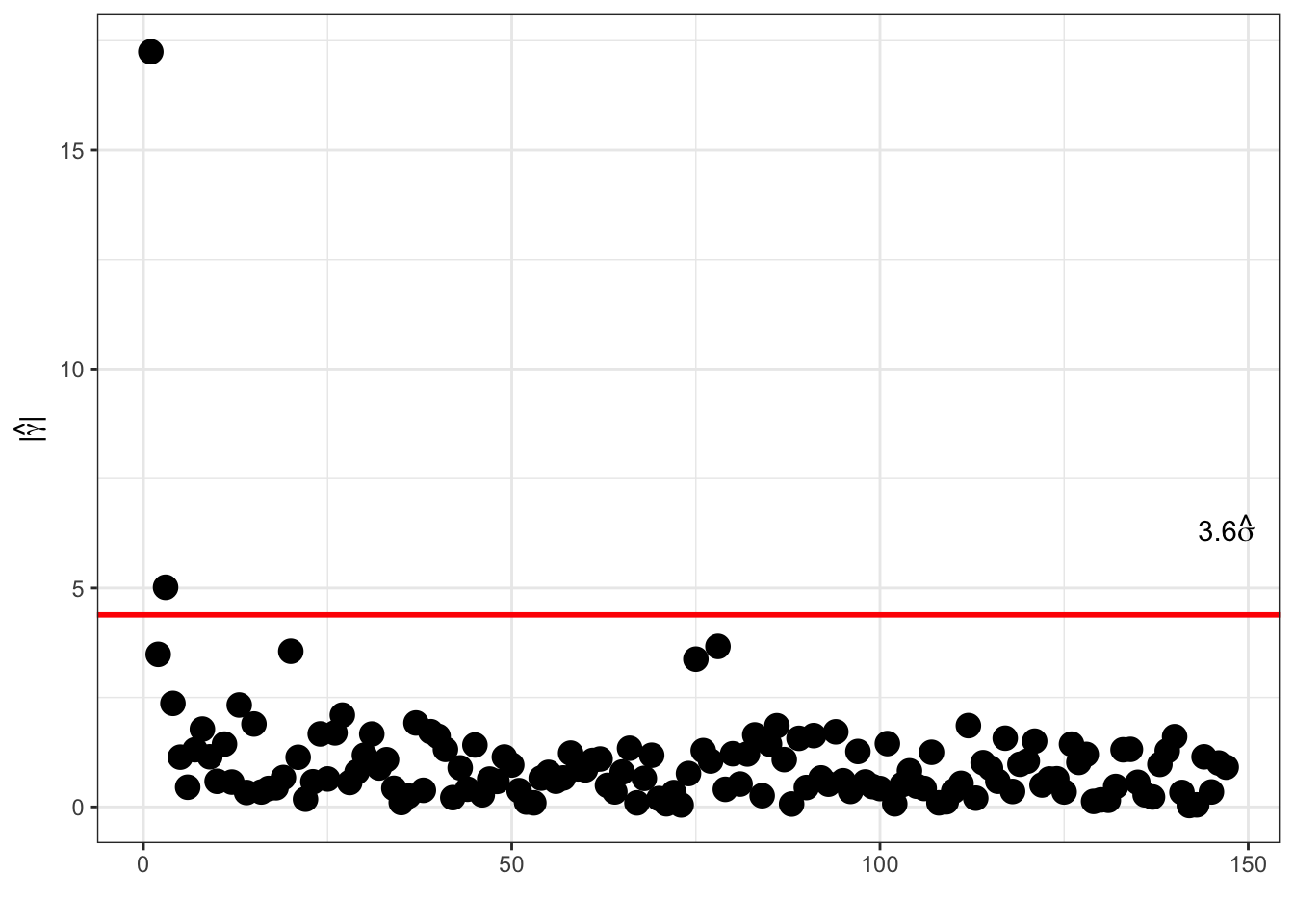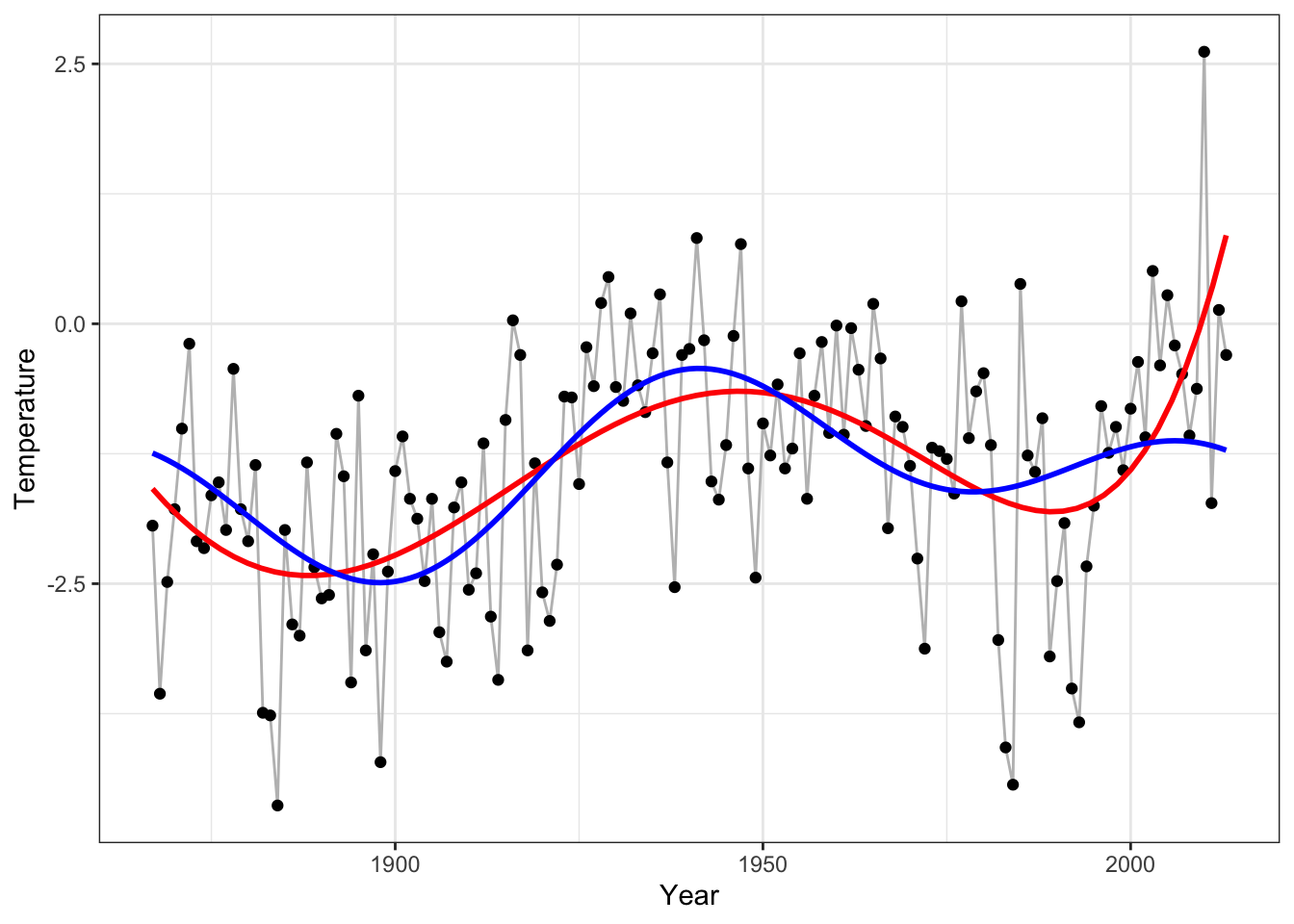Figure 3.12: Fourier based smoother by thresholding (blue) and polynomial fit of degree 5 (red).

What is the point using the discrete Fourier transform? The point is that the discrete Fourier transform can be computed via the fast Fourier transform (FFT), which has an $$O(n\log(n))$$ time complexity. The FFT works optimally for $$n = 2^p$$.

fft(Nuuk_year\$Temperature)[1:4] / sqrt(n)
##  -17.246965+0.000000i  -2.464289+2.387119i   3.548133+0.909923i   1.672144+0.741358i
betahat[1:4]
##  -17.246965+0.000000i  -2.464289+2.387119i   3.548133+0.909923i   1.672144+0.741358i